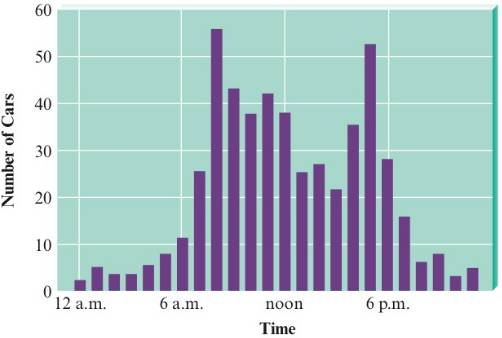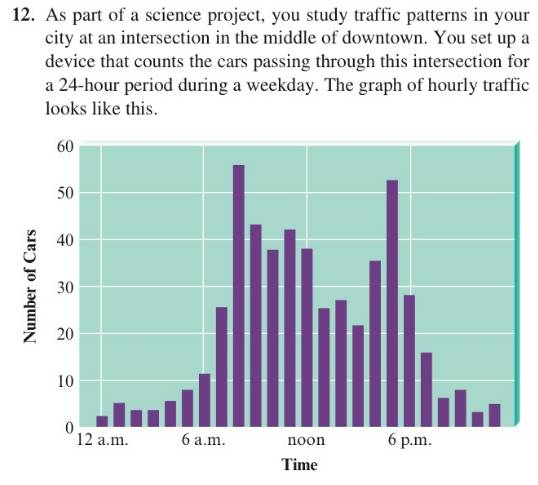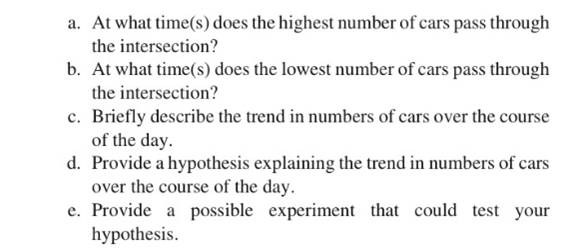# As part of a science project, you study traffic patterns in your city at an intersection in the middle of downtown. You set up a device that counts the cars passing through this intersection for a 24-hour period during a weekday. The graph of hourly traffic looks like this. At what lime(s) does the highest number of cars pass through the intersection? At what time(s) does the lowest number of cars pass through the intersection? Briefly describe the trend in numbers of cars over the course of the day. Provide a hypothesis explaining the trend in numbers of cars over the course of the day. Provide a possible experiment that could test your hypothesis.### Introductory Chemistry: A Foundati...

9th Edition
Steven S. Zumdahl + 1 other
Publisher: Cengage Learning
ISBN: 9781337399425

#### Solutions

Chapter
Section### Introductory Chemistry: A Foundati...

9th Edition
Steven S. Zumdahl + 1 other
Publisher: Cengage Learning
ISBN: 9781337399425
Chapter 1, Problem 12ALQ
Textbook Problem
264 views

## As part of a science project, you study traffic patterns in your city at an intersection in the middle of downtown. You set up a device that counts the cars passing through this intersection for a 24-hour period during a weekday. The graph of hourly traffic looks like this.At what lime(s) does the highest number of cars pass through the intersection? At what time(s) does the lowest number of cars pass through the intersection? Briefly describe the trend in numbers of cars over the course of the day. Provide a hypothesis explaining the trend in numbers of cars over the course of the day.Provide a possible experiment that could test your hypothesis.(a)

Interpretation Introduction

Interpretation:

We need to determine the time when the highest number of cars pass through the intersection.

Concept Introduction:

We study traffic patterns in a city at an intersection in the middle of downtown. The device that counts the number of cars passing through this intersection for a twenty four hour period on a weekday is set up. The graph of the hourly traffic is shown.

### Explanation of Solution

We study traffic patterns in a city at an intersection in the middle of downtown. The device that counts the number of cars passing through this intersection for a twenty four hour period on a weekday is set up. The graph of the hourly traffic is given...

Interpretation Introduction

(b)

Interpretation:

We need to determine the time at which the lowest number of cars pass through the intersection.

Concept Introduction:

We study traffic patterns in a city at an intersection in the middle of downtown. The device that counts the number of cars passing through this intersection for a twenty four hour period on a weekday is set up. The graph of the hourly traffic is shown.

Interpretation Introduction

(c)

Interpretation:

We need to describe the trend in the number of cars passing through the intersection each day.

Concept Introduction:

We study traffic patterns in a city at an intersection in the middle of downtown. The device that counts the number of cars passing through this intersection for a twenty four hour period on a weekday is set up. The graph of the hourly traffic is shown.

Interpretation Introduction

(d)

Interpretation:

We need to form a hypothesis explaining the trend in the number of cars passing through this intersection each day.

Concept Introduction:

We study traffic patterns in a city at an intersection in the middle of downtown. The device that counts the number of cars passing through this intersection for a twenty four hour period on a weekday is set up. The graph of the hourly traffic is shown.

Interpretation Introduction

(e)

Interpretation:

We need to create and perform the experiment which will test the hypothesis.

Concept Introduction:

We study traffic patterns in a city at an intersection in the middle of downtown. The device that counts the number of cars passing through this intersection for a twenty four hour period on a weekday is set up. The graph of the hourly traffic is shown. Based on the graph, we try to test the hypothesis.

### Still sussing out bartleby?

Check out a sample textbook solution.

See a sample solution

#### The Solution to Your Study Problems

Bartleby provides explanations to thousands of textbook problems written by our experts, many with advanced degrees!

Get Started

Find more solutions based on key concepts
To overload a muscle is never productive. T F

Nutrition: Concepts and Controversies - Standalone book (MindTap Course List)

Why are interstellar lines so narrow?

Horizons: Exploring the Universe (MindTap Course List)

Where is an ignition signal located?

Introduction to General, Organic and Biochemistry

Complete the following table:

Foundations of Astronomy (MindTap Course List)

Which type of sediment is rarest? Where does this sediment originate?

Oceanography: An Invitation To Marine Science, Loose-leaf Versin

Let the polar coordinates of the point (x, y) be (r, ). Determine the polar coordinates for the points (a) (x, ...

Physics for Scientists and Engineers, Technology Update (No access codes included)

Why is it more difficult to determine the sex of a newly hatched canary than a newborn puppy?

Biology: The Unity and Diversity of Life (MindTap Course List)